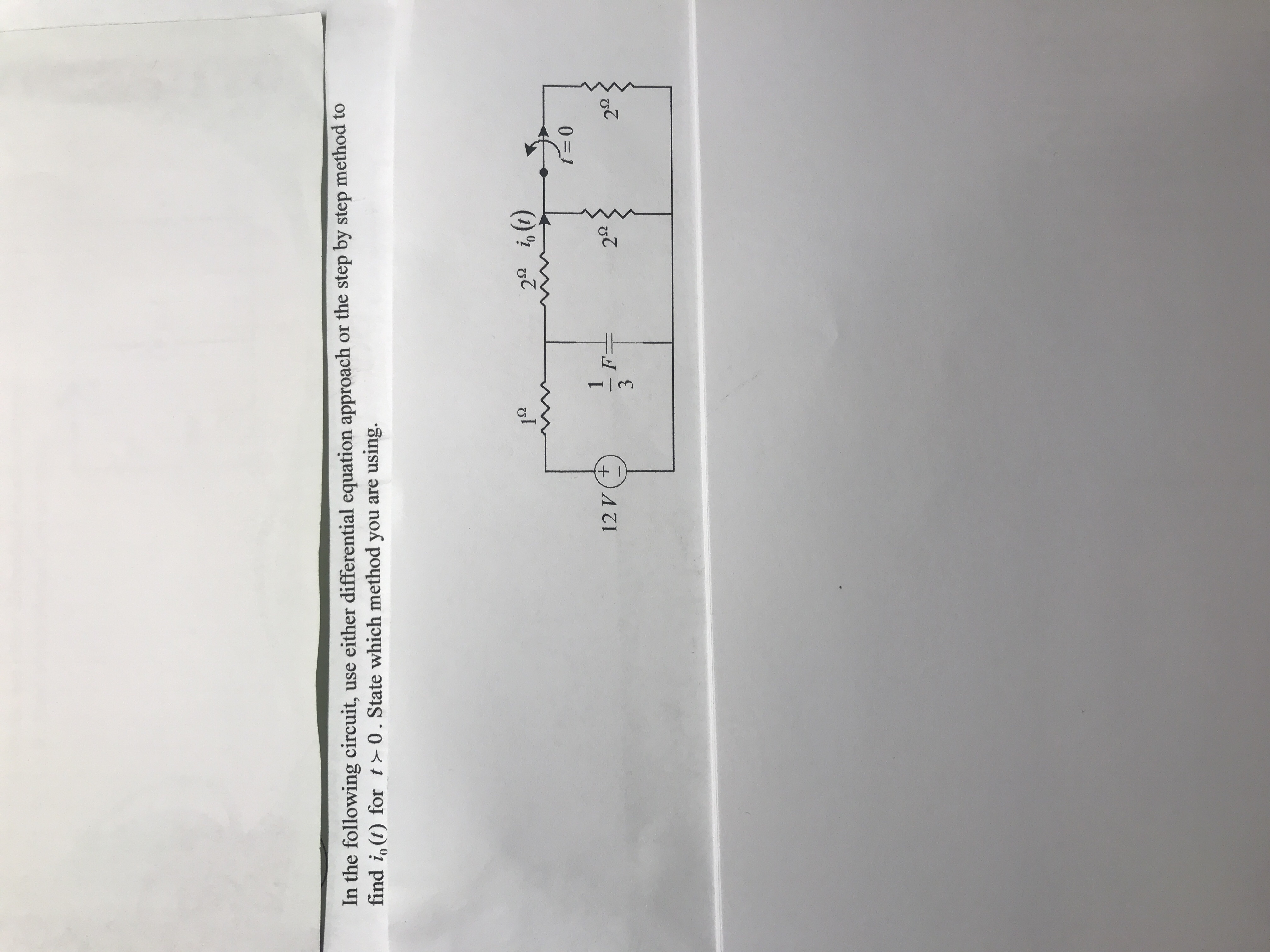# In the following circuit, use either differential equation approach or the step by step method tofind i。(t) for t 0 . State which method you are using.1s229る(1)12 V(+2Ω2Ω

Question
27 viewshelp_outlineImage TranscriptioncloseIn the following circuit, use either differential equation approach or the step by step method to find i。(t) for t 0 . State which method you are using. 1s2 29 る(1) 12 V(+ 2Ω 2Ω fullscreen
check_circle

Step 1

STEP BY STEP METHOD:

From the given circuit, the switch opens at t =0. That is, switch is in closed condition before t =0. The capacitor gets open circuited at steady-state condition. Therefore, draw the circuit for t =0- (just before open the switch) as shown in Figure 1 and find the value of voltage across the capacitor just before open the switch.

Step 2

From Figure 1, the resistors 2 ohms and 2 ohms are in parallel. The equivalent resistance of two 2 ohms resistors is 1 ohm. Then, the equivalent 1 ohm resistance is connected in series with the 2 ohm resistor. Therefore, the equivalent resistance of 1 ohm and 2 ohm resistor is 3 ohms. Now, the equivalent resistance across the capacitor is 3 ohms.

Consider the analysis from Figure 1, and apply the voltage division rule to find the voltage across capacitor for t =0- as follows.

Step 3

As the switch is in open condition for t =0, the switch continues to be in open condition for t =0+. Therefore, redraw the circuit for t ...

### Want to see the full answer?

See Solution

#### Want to see this answer and more?

Solutions are written by subject experts who are available 24/7. Questions are typically answered within 1 hour.*

See Solution
*Response times may vary by subject and question.
Tagged in

### Electrical Engineering